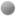rejetto forum### [upload name] and {.from table.}

Mars · 3 · 2148

0 Members and 1 Guest are viewing this topic.

####Mars

• Operator
• Tireless poster
•• Posts: 2039
one bug with [upload name] event
http://www.rejetto.com/forum/index.php?topic=7097.0

one other with {.from table.}

Quote
function fromTable(tbl, k:string):string;
...
if tbl = 'ini' then deprecatedMacro('from table|ini','from table|#ini');
....
if (tbl = 'ini') and (result = 'no') then result:=''; // we are reading a value from the ini, so we convert the 'no' to a valid false value (the empty string)
end; // fromTable
The code is wrong if we want to use the new shape with #ini, this line is never executed, it must be replaced by:

if stringExists(tbl, ['ini','#ini']) and (result = 'no') then result:='';

« Last Edit: January 25, 2010, 10:49:49 PM by Mars »

####Mars

• Operator
• Tireless poster
•• Posts: 2039
I take advantage of this opportunity to propose an extension for the macro {.from table.}

Just a small reminder about the wiki:

Quote
from table | A | B
A is the table and B is the key. The table is a variable or a section with this form
key1=value1
key2=value2
key3=value3
Let's say such text is the content of variable foo, then to get value2 you should do {.from table|foo|key2.}. (yes, poetry)
If it was the content of table [bar] instead, then you should do
{.from table|\$bar|key2.}.

The quite last line in blue allows to get back the value of a specific line in a table contained in a section of the main template like by eg:

[bar]
key1=texte1
key2=texte2
key3=texte3

The only marigold is that it is imperative that the section is in the main template. I brought a modification which is in board 'programmers corner' and which allows for this syntax to specify the file source.

the macro is {.from table|\$bar|key2|file=xxxxxxx.}

file=word.txt                  word.txt into the hfs.exe path
file=languages/word.txt    word.txt into a subdir of the hfs.exe path
file=/template/word.txt     word.txt into the VFS   ( this line is to the RAWR)
file=c:\........\word.txt      word.txt into a drive

if one of characters / or \  are into the line file=xxxx , then you have to dequote the result if key2 contain some macros (it's a security used into the macro {.section.} and taken back here)

Quote

function fromTable(tbl, k:string):string;     //mars
var
t: Ttpl;
i: integer;
space, h: THashedStringList;
s: string;
begin
result:='';
if tbl = 'ini' then deprecatedMacro('from table|ini','from table|#ini');
space:=getVarSpace(tbl);
if not satisfied(space) then exit;
if pars.indexOfName('file') < 0 then i:=space.indexOfName(tbl) else i:=-1;
if (i < 0) and ansiStartsStr('\$', tbl) then
begin
if pars.indexOfName('file') < 0 then
s:=md.tpl[copy(tbl,2,MAXINT)]
else begin
t:=Ttpl.create;
try
s:=t[copy(tbl,2,MAXINT)];
finally t.free end;
end;

if s = '' then exit;
end;
if i < 0 then exit;
// the text of the table is converted to a hashed structure, and cached through the objects[] property
h:=space.objects[i] as THashedStringList;
if h = NIL then
begin
h:=ThashedStringList.create();
h.text:=space.valueFromIndex[i];
space.objects[i]:=h;
end;
result:=h.values[k];
// templates outside hfs folder get quoted for security reasons
if anyCharIn('/\', par('file')) then
result:=macroQuote(result);

if stringExists(tbl, ['ini','#ini']) and (result = 'no') then result:=''; // we are reading a value from the ini, so we convert the 'no' to a valid false value (the empty string)  end; // fromTable« Last Edit: August 17, 2009, 09:53:15 PM by Mars »

####rejetto

• Tireless poster
•• Posts: 13308
thanks for reporting the bug mars,

about the "new feature", i don't think it's useful, because you can already do

{.set|bar|{.section|bar|file=external.txt.}.}
...
{.from table|bar|key.}

or a much faster way

{.if not|{.^#bar.}|{:
{.set|#bar|{.section|bar|file=external.txt.}.}
:}.}
...
{.from table|#bar|key.}